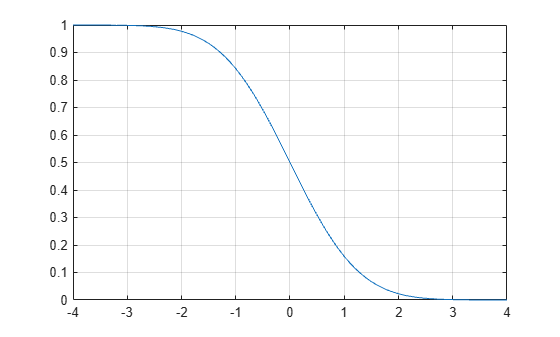Documentation

### This is machine translation

Mouseover text to see original. Click the button below to return to the English version of the page.

# qfunc

## Syntax

``y = qfunc(x)``

## Description

example

````y = qfunc(x)` returns the output of the Q function for each element of the real array `x`. The Q function is one minus the cumulative distribution function of the standardized normal random variable.```

## Input Arguments

collapse all

Input, specified as a real scalar or array.

## Output Arguments

collapse all

Q function output, returned as a real scalar or array having the same dimensions as input `x`.

## Examples

collapse all

Determine the values of the Q function for an input vector.

```x = -4:0.1:4; y = qfunc(x);```

Plot the results.

```plot(x,y) grid```Convert an input Eb/No in dB to its linear equivalent.

```ebnodB = 7; ebno = 10^(ebnodB/10);```

Determine the QPSK error probability, ${P}_{b}$, given that:

`${P}_{b}=Q\left(\sqrt{2\frac{Eb}{No}}\right).$`

`Pb = qfunc(sqrt(2*ebno))`
```Pb = 7.7267e-04 ```

## Algorithms

For a scalar `x`, the formula is

`$Q\left(x\right)=\frac{1}{\sqrt{2\pi }}\underset{x}{\overset{\infty }{\int }}\mathrm{exp}\left(-{t}^{2}/2\right)dt$`

The Q function is related to the complementary error function, erfc, according to

`$Q\left(x\right)=\frac{1}{2}\text{erfc}\left(\frac{x}{\sqrt{2}}\right)$`